## Shift the contour of integration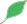This article is a stub.This means that it cannot be considered to contain or lead to any mathematically interesting information.

### Quick description

When integrating a holomorphic (or meromorphic) function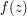on some contour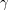in the complex plane (or even just on an interval in the real line, such as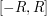), bear in mind that Cauchy's integral theorem and its variants (such as the Cauchy integral formula and the residue theorem are available to shift the contourof integration to a different contour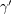with the same endpoints; the above theorems will, in many cases, guarantee that the two contour integrals are the same, except possibly for some correction terms coming from poles or other singularities betweenand.

This technique is often good for bounding integrals, because one can sometimes shift the contour into regions where the integrand (and hence the integral) is quite small. In some cases, one can even shift the contour all the way to infinity by an appropriate limiting process, which can allow one to use complex methods to evaluate a number of improper integrals exactly.

Complex analysis

### Example 1

(Maybe do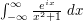in detail? Note that Jordan's lemma is already discussed at base times height and Bound by a Riemann sum.)

### General discussion

It is important to note that contour shifting methods generally only work when the integrand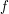can be expressed as a holomorphic (or at least meromorphic) function of the variableof integration. In particular, integrands involving expressions such as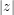or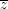tend to not be amenable to contour integration techniques, unless the integrand can somehow be rewritten to eliminate such expressions.

A classical application of the contour shifting method to bound integrals is the method of steepest descent.